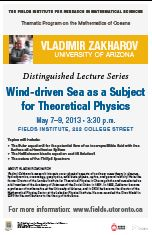THEMATIC PROGRAMSJune 25, 2019THE FIELDS INSTITUTE FOR RESEARCH IN MATHEMATICAL SCIENCES
20th ANNIVERSARY YEARApril 29-June 28, 2013 Thematic Program on the Mathematics of Oceans Coxeter Lecture Series May 7,9,10, 2013 at 3:30 p.m. Fields Institute, 222 College St. Toronto Vladimir Zakharov University of Arizona Wind-driven Sea as a Subject for Theoretical PhysicsMay 07, 2013 at 3:30 pm. Lecture 1. We will show that the Euler equations for potential flow of incompressible fluid with free surface is a Hamiltonian system. The canonical variables are the surface elevation and the potential evaluated on the surface. Instead of these ”natural variables” we can introduce normal canonical variables, which are classical analogs of annihilation and creation operators in the quantum field theory. This language is very convenient. Then one can perform the canonical transformation excluding the cubic terms in the Hamiltonian. The resulting effective Hamiltonian describes scattering of the ”riplons” and conserves the total wave action. The coupling coefficient for nontrivial scattering of riplons in 2D geometry happens to be identically zero. This fact makes possible the further simplification of the Hamiltonian. The resulting ”compact Hamiltonian” describes interaction of riplons moving in one direction. From this Hamiltonian, the Nonlinear Schrodinger equation and the Dysthe equation can be obtained in the case of narrow frequency band. The ”compact” four-wave equation is very suitable for numerical simulation. It admits solitonic solution that is very similar to the rogue waves observed in the ocean. May 09, 2013 at 3:30 pm. Lecture 2 on Hasselmann kinetic equation and its solutions Using the four-riplon Hamiltonian one can easily derive the Hasselmann kinetic equation for the wave action spectrum. This equation is almost identical to the standard kinetic equations for phonons in the condensed matter physics. The Hasselmann kinetic equation has remarkable powerlike solutions of Kolmogorov type that describe the direct cascade of energy and the inverse cascade of wave action. These solutions are observed in laboratory and field experiments as well as in numerical simulation of primordial deterministic equations. The Hasselmann kinetic equation admits very broad class of self-similar solutions that describe the dependence of major characteristics of wind-driven sea: the average energy and the mean frequency on duration and fetch. The use of selfsimilar solutions makes possible to explain the results of numerous field and laboratory experiments. They show the domination of four-riplon scattering over other processes: interaction with the wind and dissipation due to wave breaking. This fact is supported by the direct numerical solution of the kinetic equation. May 10, 2013 at 3:30 pm. Lecture 3. About the nature of Phillips spectrum Numerous experiments show that the KZ spectrum $I(\omega)\simeq \omega^{-4}$ is realized in a limited (one decade) range of wave numbers right behind the spectral peak. More short waves obey to more steep Phillips law $I(\omega)\simeq g^2 \,\omega^{-5}$. This spectrum can be obtained from dimensional consideration (O. Phillips 1957). From the beginning, it was clear that existence of this spectrum is connected with wave breaking event but its physical nature was understood just recently. Each breaking event is defined by a characteristic length, while the Phillips spectrum includes one dimension quantity only, the gravity acceleration $g$. This contradiction is resolved as follow. Existence of Phillips spectrum presumes coexistence of plethora of wave breaking events with different characterizing scales. Moreover, there is equipartition of inverted wave breaking scales. Simultaneous presence of the weak turbulent KZ spectrum $\omega^{-4}$ and the Phillips spectrum $\omega^{-5}$ means that in the real sea coexist both the weak and the strong wave turbulence. Long waves weakly interact due to four-wave resonant processes. This interaction forms the direct cascade of energy that feeds the wave breaking mechanism. Also, the four-wave interaction creates the inverse cascade, the frequency downshift. Weakly interacting long waves are covered by the net of microscopic wave-breakings. Including into kinetic equation an additional term of very sophisticated form, we can take into account the wave-breaking event.

Biography:
After completing his Candidate of Science degree, Zakharov worked as a researcher at the Budker Institute of Nuclear Physics in Novosibirsk, where in 1971 he completed his Doctor of Sciences degree. In 1974, Zakharov moved to the Landau Institute for Theoretical Physics in Chernogolovka, where he eventually became director. He was elected as a corresponding member of the Academy of Sciences of the Soviet Union in 1984 and as a full member in 1991. In 1992, Zakharov became a professor of mathematics at the University of Arizona, and in 2004 he became the director of the Mathematical Physics Sector at the Lebedev Physical Institute.

Speakers in the Coxeter Lecture Series (CLS) have made outstanding contributions to their field of mathematics. The CLS consists of a series of three one-hour lectures.